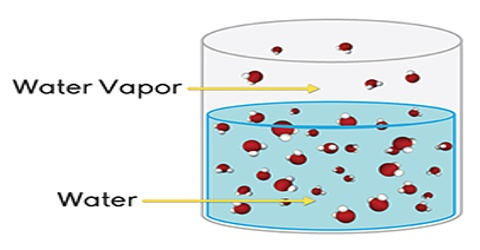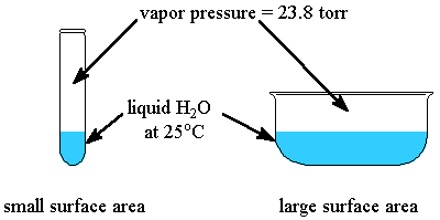# Lowering of Vapour Pressure: Properties of Dilute Solutions

Lowering of Vapour Pressure: Properties of Dilute Solutions

If a non-volatile and non-electrolyte solute like sucrose, glucose or urea is dissolved in a solvent like water the vapour pressure of the solution is found to be lower than that of pure water. The magnitude of the lowering of vapour pressure is dependent on the concentration of the solute in solution. Although attempts had been made to relate vapour pressure lowering quantitatively to concentration since the days of Berthollet and Faraday, the credit for stating the correct relationship goes to F.M. Raoult (1837-1888). He stated that the relative lowering of vapour pressure of a solvent due to the addition of a non-volatile and non-electrolyte solute is equal to the mole fraction of the solute in the solution. This statement, which is known as Raoult’s law, is an important generalization in Physical Chemistry and is fundamental to the study of solutions.Relative lowering is defined as the ratio of the difference of vapour pressures of the pure solvent and the solution to the vapour pressure of the pure solvent. The mole fraction of the solute on the other hand is the ratio of the number of moles of the solute to the total number of moles in solution. If p0 and p are vapour pressures of the solvent and the solution respectively, the lowering of vapour pressure is (p° – p), and relative lowering of vapour pressure is [(p° – p)/p0]. In a solution containing, n moles of solute in N moles of solvent the mole fraction of the solute is n/(N + n). Then according to Raoules law,

(p° – p)/p0 = n / (N+n) = x2

where x2 is the mole fraction of the solute. Experimental supports in favor of the law have been obtained by various investigators. The law it however, valid only for dilute solutions of solutes which do not undergo association or disassociation in the dissolved state.